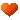IKO LWL7C2R250BHS2直线导轨

H=8                                                     h=2.3
H1=1.5                                                E=7.5
N=5                                                     F=15

W2=17                                                W3=12
W4=2.5                                               L1=23.5
L3=14.3                                              区间价格：450.0
~1200.0（H/P)服务热线⇒13916112065